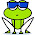## Search This Blog

bc utility is surely one of the underdogs when it comes to calculations on command line.
It’s a feature rich utility that has its own fan base but needs time to get comfortable with
• bc is included with (almost?) all Linux distros as standard, as well as (again, almost?) all Unices.
• Results from calculations in some proprietary flavours of `bc` have up to 99 decimal digits before and after the decimal point. This limit has been greatly surpassed in GNU `bc`. I don't know what that limit is, but it's at least many, many tens of thousands. Certainly it's more than any GUI-based calculators (I've used) could accomodate.
• You may also find yourself working in an environment where you simply don't have access to a GUI.
• The syntax for basic sums is almost identical to Google's calculator function, so you can learn how to use two utilities in one go!
bc is a preprocessor for dc. The useful thing about bc is that it accepts input from files and from standard input. This allows us to pipe data to it for quick calculations.

basic calculations example
bc <<< 5*4
20
bc <<< 5+4
9
bc <<< 5-4
1
So you can see that it’s so very easy to do basic calculations with bc.
There is another way of doing the same by using echo command.
Here are some examples:
\$ echo "5*4" | bc
20
\$ echo "5+4" | bc
9
\$ echo "5-4" | bc
1
So you can see that, for simple and quick calculations, just use echo and pipe it to bc command.
NOTE – If you have some expressions in a file that are ready to be calculated then you can also use bc to calculate those expressions. Here is an example :
\$ cat calcFile
5+5
6+7
\$ bc < calcFile
10
13
To use the result of last calculation within the same expression that is passed to bc
\$ echo "5-4;last+6" | bc
1
7
need more ? what to get pro with it ? here's some more examples

scale
The scale variable determines the number of digits which follow the decimal point in your result. By default, the value of the scale variable is zero. (Unless you use the -l option in which case it defaults to 20 decimal places. More about -l later.) This can be set by declaring scale before your calculation, as in the following division example:
division
\$ echo 'scale=25;57/43' | bc

1.3255813953488372093023255

square root

\$ echo 'scale=30;sqrt(2)' | bc

1.414213562373095048801688724209
This beats Google's calculator function which only calculates the result to 8 decimal places! ;-) Although Google's calculator function has this 8 decimal places limitation, it will allow imaginary numbers as answers. re ? what to get pro with it ? here's some more examples
power
\$ echo '6^6' | bc

46656
parentheses
If you have read Robert Heinlein's The Number of the Beast, you may recall that the number of parallel universes in the story equals (six to the power of six) to the power of six. If you should try to calculate that like this:
\$ echo '6^6^6' | bc
You will get a screen full of numbers (some 37374 digits), not the
10314424798490535546171949056
that you might expect.

obase and ibase
obase and ibase are special variables which define output and input base.
Legitimate obase values range from 2 to 999, although anything beyond 16 is wasted on me!
Legitimate ibase values range from 2 to 16.
Some examples will explain all this better.
Here we're converting 255 from base 10 to base 16:
\$ echo 'obase=16;255' | bc
FF
convert from decimal to binary
And here we're converting the number 12 from base 10 to base 2:
\$ echo 'obase=2;12' | bc
1100
Which reminds me of the old joke:
There are only 10 types of people in the world -- those who understand binary, and those who don't.
Which leads us neatly onto the next example:

convert from binary to decimal
Here we're converting the binary number 10 to a base 10 (decimal) number.
\$ echo 'ibase=2;obase=A;10' | bc
2
Note that the obase is "A" and not "10". Sorry, you've got to learn some hex. The reason for this is you've set the ibase to "2", so if you now had tried to use "10" as the value for the obase, it would stay as "2", because "10" in base 2 is "2". So you need to use hex to "break out" of binary mode.

Well, that was just to explain the joke; now something a bit more challenging:
\$ echo 'ibase=2;obase=A;10000001' | bc
129
\$ echo 'ibase=16;obase=A;FF' | bc
255
Again, note the use of "A" to denote base 10. That is because "10" in hex (base 16 - the ibase value) is 16.

There is much more to do with the bc commend and you will learn more as you start using it :)Share it:

#### Linux Commands

1.